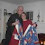## Sunday, December 17, 2006

### A Quiet Day on the Island

About 20 minutes ago I received the following puzzle in my email. It's quiet on the island today so I thought it would be fun to mess about with it. If you've got a few minutes for a puzzle read on.

Post a comment if you want to explain the puzzle. I've already posted my explanation. Just click on "comments" below if you want to post or peek. (It really is quiet here today!!)

AGE BY DINER & RESTAURANT MATH

DON'T CHEAT BY SCROLLING DOWN FIRST!
It takes less than a minute .
Work this out as you read .
Be sure you don't read the bottom until you've worked it out!

1. First of all, pick the number of times a week that you would like to go out to eat. (more than once but less than 10)
2. Multiply this number by 2 (just to be bold)
4. Multiply it by 50
6. Now subtract the four digit year that you were born. You should have a three digit number

The first digit of this was your original number
(i.e., how many times you want to go out to restaurants in a week.)
The next two numbers are YOUR AGE! (Oh YES, it is!!!!!)

THIS IS THE ONLY YEAR (2006) IT WILL EVER WORK, SO SPREAD IT AROUND WHILE IT LASTS."

.

1.It's algebra.

Given 3 as an example of the answer to how many days do you want to eat out, this is the formula:
((3 x 2) + 5) x 50 [this is what the puzzle asks you to do, namely choose a number between 1 & 10, double it, add 5, then multiply it by 50]. This is algebraically equivalent to (3 x 2 x 50) + (5 x 50) which equals (3 x 100) + 250 which equals 300 + 250.

When you add 1756 and 250 (the 250 in the answer above, derived by multiplying 5 x 50) you get the current year, 2006. There is no mystery to that.

Subtract your birth year, and of course you get your current age.

By factoring in the other 100 (derived by multiplying 2 x 50) and then multiplying by the first number chosen (3 in this example) you get that number (3) in the hundreds place of your final answer.

It will work for other years, not just 2006. For years from now to 2009 simply substitute another appropriate number for 1756. For example, next year the puzzle would ask you to choose a number (this time we'll choose 5), multiply by 2 (2 x 5 = 10); add 5 (10 + 5 = 15), multiply by 50 (15 x 50 = 750); add 1757 (not 1756) this time (750 + 1757 = 2507); now I subtract the year of my birth (2507 - 1944 = 563). So I now get 5 (the number I first chose) and 63, my age in Dec. 2007. I haven’t done all the math, but other numbers & other calculations would have to be substituted once we reach 2010.

For example in the year 2017, 70 could be substituted for 50, and 1747 could be substituted for 1756. And we’d have to subtract 200 somehow (e.g. by changing step #4 to “Multiply it by 70, then subtract 200”). So the formula we’d get would be ((3 x 2) + 5) x 70 = 770. 770 – 200 = 570. 570 + 1747 = 2317. 2317 - 1944 = 373. Other years would require other, slightly different formulas.

2.Just for clarification, the last paragraph of my explanation assumes wanting to eat out 3 times per week, and a birth date of 1944.

3.I should have left well enough alone! My example for 2017 works for the number 3, but not for 5, e.g. I'm sure there are workable formulas for these other years, but I think I'll just go home and read a book. I'm not inspired to mess around with this any longer.

Maybe another reader wants to tackle it. And maybe not.

4.Anonymous4:43 PM

Philip,
How cool is this puzzle? By the way, the bunting was absolutely beautiful!! It didn't look real, but I know it was. Have a Merry Christmas. Carol in Ohio